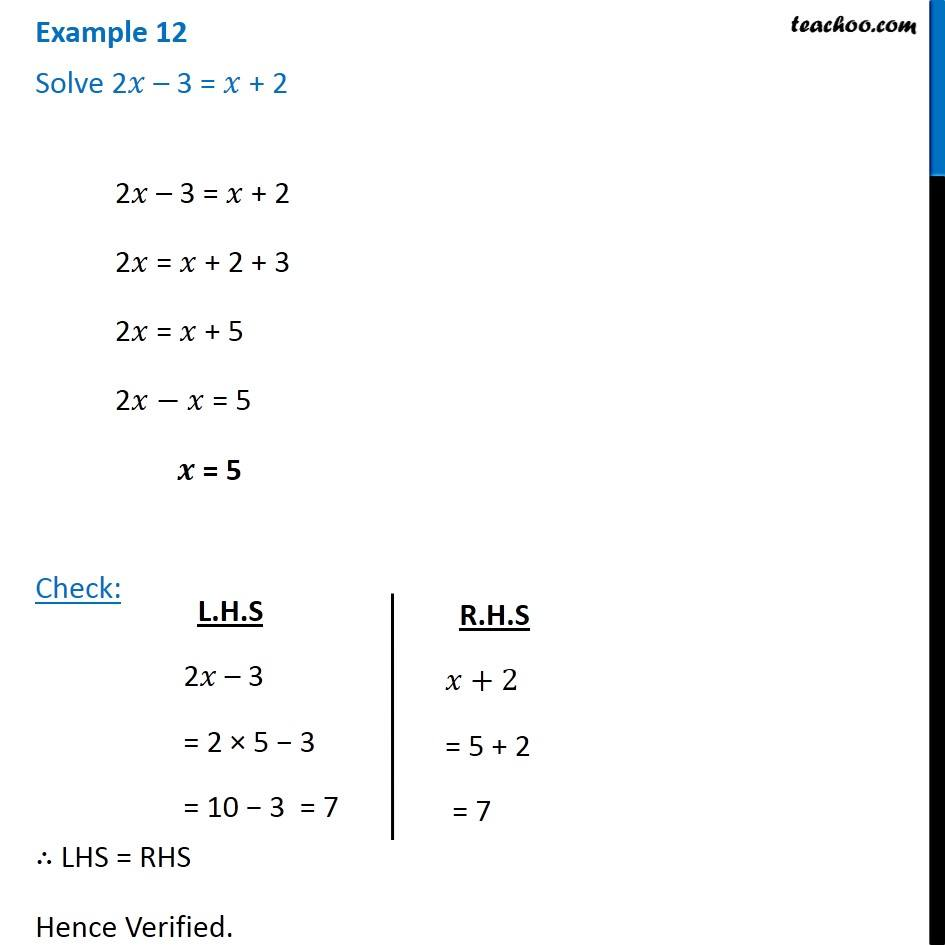1. Chapter 2 Class 8 Linear Equations in One Variable
2. Concept wise
3. Solving Linear Equations - Variables on both sides

Transcript

Example 12 Solve 2 3 = + 22 3 = + 2 2 = + 2 + 3 2 = + 5 2 = 5 = 5 Check: LHS = RHS Hence Verified. L.H.S 2 3 = 2 5 3 = 10 3 = 7 R.H.S +2 = 5 + 2 = 7